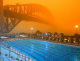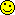# Math Is Fun Forum

Discussion about math, puzzles, games and fun.   Useful symbols: ÷ × ½ √ ∞ ≠ ≤ ≥ ≈ ⇒ ± ∈ Δ θ ∴ ∑ ∫  π  -¹ ² ³ °

You are not logged in.

## #1 2023-10-31 09:19:38

iiooasd1217
Novice
Registered: 2023-10-31
Posts: 5

### Fibonacci Number and Perfect Squares

This is a puzzle I have been thinking about more than I would like, so I thought I could share it. How many perfect squares are in the Fibonacci sequence? This problem can be extended to other P-Fibonacci sequences and the tribonacci. Here's a hint. 1 and 144 are two examples of perfect squares in the Fibonacci sequence.

I'll do a^2+b^2=c^2. I'll even do pir^2. But y=mx+b is where I draw the line.
Why do people become maths teachers? Because they have problems.

Offline

## #2 2023-11-01 18:17:41

phrontister
Real MemberFrom: The Land of Tomorrow
Registered: 2009-07-12
Posts: 4,793

### Re: Fibonacci Number and Perfect Squares

iiooasd1217 wrote:

How many perfect squares are in the Fibonacci sequence?

Hi iiooasd1217,

I could only find 0, 1 and 144 in the first 100,000 Fibonacci numbers.

144 is the 13th Fibonacci number (sequence A000045 in the OEIS).

My Mathematica code:

``````In:= AbsoluteTiming[Select[Sqrt@LinearRecurrence[{1,1},{0,1},100000],IntegerQ]^2]
Out= {5206.4986953,{0,1,1,144}}``````

Computing speed with that code is about the same as with M's Fibonacci function: Table[Fibonacci[n], {n, 100000}].

Testing to 1M would tie up my computer for longer than I'd like, so I'll quit now.

Also, extending my test range may be a fruitless exercise, as in his article Square Fibonacci Numbers, Etc, John H. E. Cohn 'apparently' proves that there are no more results than just the three I found.

I say 'apparently', because his proof is way beyond my understanding.The article begins:
"An old conjecture about Fibonacci numbers is that 0, 1 and 144 are the only perfect squares. Recently there appeared a report that computation had revealed that among the first million numbers in the sequence there are no further squares . This is not surprising, as I have managed to prove the truth of the conjecture..."

Last edited by phrontister (2023-11-06 14:06:30)

"The good news about computers is that they do what you tell them to do. The bad news is that they do what you tell them to do." - Ted Nelson

Offline

## #3 2023-11-06 10:25:33

phrontister
Real MemberFrom: The Land of Tomorrow
Registered: 2009-07-12
Posts: 4,793

### Re: Fibonacci Number and Perfect Squares

iiooasd1217 wrote:

How many perfect squares are in the Fibonacci sequence? This problem can be extended to ... the tribonacci.

Hi iiooasd1217,

I only found six solutions (0, 1, 4, 81, 3136 and 10609) in the first 100,000 tribonacci numbers.

10609 is the 19th tribonacci number (sequence A000073 in the OEIS).

And as with the Fibonacci problem, I called a halt in my search at 100,000.

My Mathematica code:

``````In:= AbsoluteTiming[Select[Sqrt@LinearRecurrence[{1,1,1},{0,0,1},100000],IntegerQ]^2]

Out= {7393.1776884,{0,0,1,1,4,81,3136,10609}}``````

Last edited by phrontister (2023-11-06 15:03:52)

"The good news about computers is that they do what you tell them to do. The bad news is that they do what you tell them to do." - Ted Nelson

Offline

## #4 2023-11-16 15:41:50

iiooasd1217
Novice
Registered: 2023-10-31
Posts: 5

### Re: Fibonacci Number and Perfect Squares

I just wanted to say thank you for looking into this. I am an avid coder but sometimes I need help! This is so much fun to see other people looking into problems that I have been thinking about for some time now.

I'll do a^2+b^2=c^2. I'll even do pir^2. But y=mx+b is where I draw the line.
Why do people become maths teachers? Because they have problems.

Offline